Next: Example 4 Up: Examples Previous: Example 2   Contents

Example 3

This example is derived from example 2. We notice that in the three equations of example 2 the second degree terms of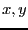are for all functions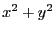. Thus by subtracting the first function to the second and third we get a linear system in. This system is solved and the value ofare substituted in the first function. We get thus a system of one equation in the unknown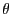(see section 15.1.2). The roots of this equation are 0,-0.8067834380. The test program is Test_Solve_Gradient_General2. The IntervalGradient function is written as:

INTERVAL_MATRIX IntervalGradient (int l1,int l2,INTERVAL_VECTOR & in)
{
25912.0+(-39320.0+14520.0*Cos(in(1)))*Cos(in(1)))*Sin(in(1));
}
With epsilonf=0 and epsilon=0.001 we get the solution by using 8 boxes: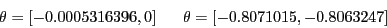for whatever order. The solution intervals contain the roots of the equation. If we use epsilon=0 and epsilonf=0.1 we get by using 8 boxes: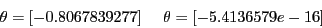Here we get a unique solution and a range solution. But we notice that the solution 0 is exactly the middle point of the test domain: Moore test will fail as 0 will always be an end-point of the range. If we break the symmetry of the test domain we will get exactly both solutions.Next: Example 4 Up: Examples Previous: Example 2   Contents
Jean-Pierre Merlet 2012-12-20